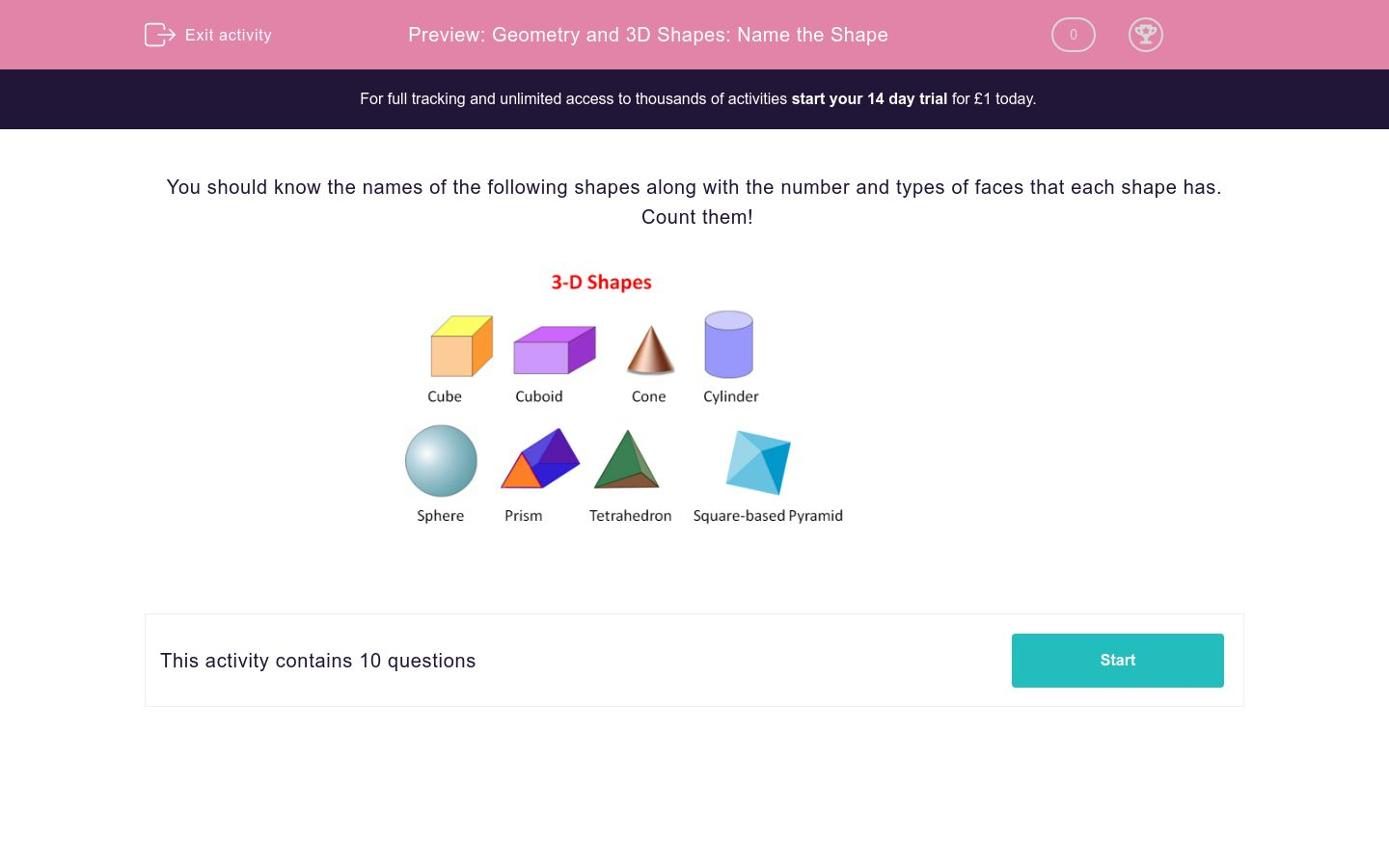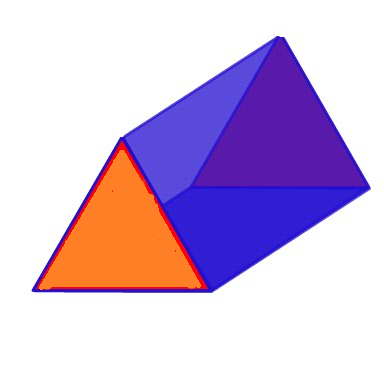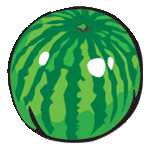# Geometry and 3D Shapes: Name the Shape

In this worksheet, students are given clues that help them name a 3D shape.Key stage:  KS 2

Curriculum topic:   Maths and Numerical Reasoning

Curriculum subtopic:   3D Shapes

Difficulty level:### QUESTION 1 of 10

You should know the names of the following shapes along with the number and types of faces that each shape has.  Count them!What is the name of a shape with.....?

6 square faces

What is the name of a shape with.....?

6 rectangular faces

What is the name of a shape which is like a.....?

Football

What is the name of a shape which is like a.....?

Toilet Roll

What is the name of a shape with.....?

A square base and a pointed top

Cone

Square-based pyramid

Tetrahedron

What is the name of a shape with.....?

4 triangular faces

Triangle

Cone

Tetrahedron

What is the name of a shape which has a.....?

Circular base and pointed top

What is the name of this shape?What shape is this watermelon roughly?Circle

Sphere

This is a picture of a mountain in Switzerland called The Matterhorn.

What shape is it roughly?Triangle

Pyramid

Cone

• Question 1

What is the name of a shape with.....?

6 square faces

Cube
• Question 2

What is the name of a shape with.....?

6 rectangular faces

Cuboid
• Question 3

What is the name of a shape which is like a.....?

Football

Sphere
• Question 4

What is the name of a shape which is like a.....?

Toilet Roll

Cylinder
• Question 5

What is the name of a shape with.....?

A square base and a pointed top

Square-based pyramid
• Question 6

What is the name of a shape with.....?

4 triangular faces

Tetrahedron
• Question 7

What is the name of a shape which has a.....?

Circular base and pointed top

Cone
• Question 8

What is the name of this shape?Prism
• Question 9

What shape is this watermelon roughly?Sphere
• Question 10

This is a picture of a mountain in Switzerland called The Matterhorn.

What shape is it roughly?